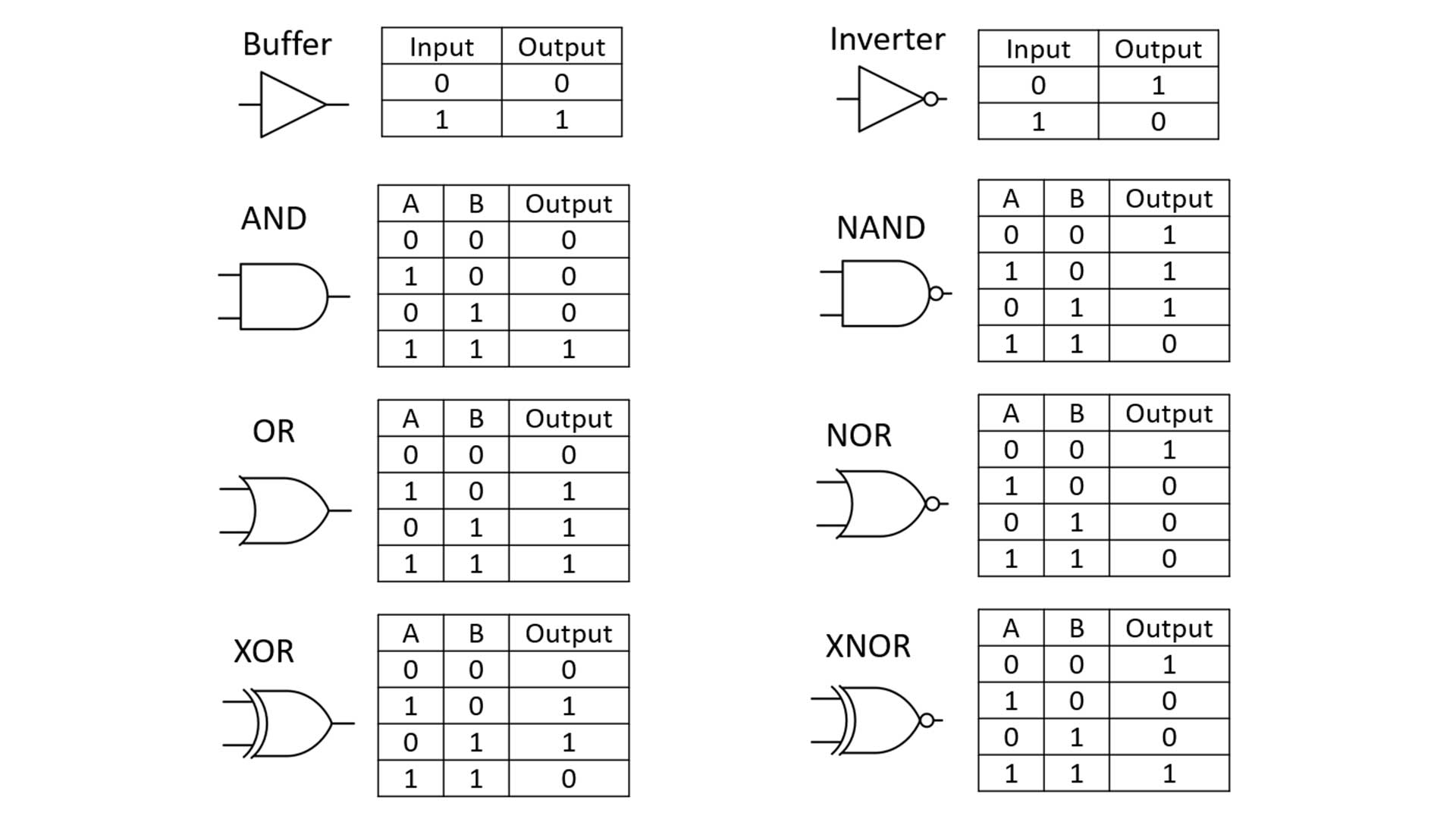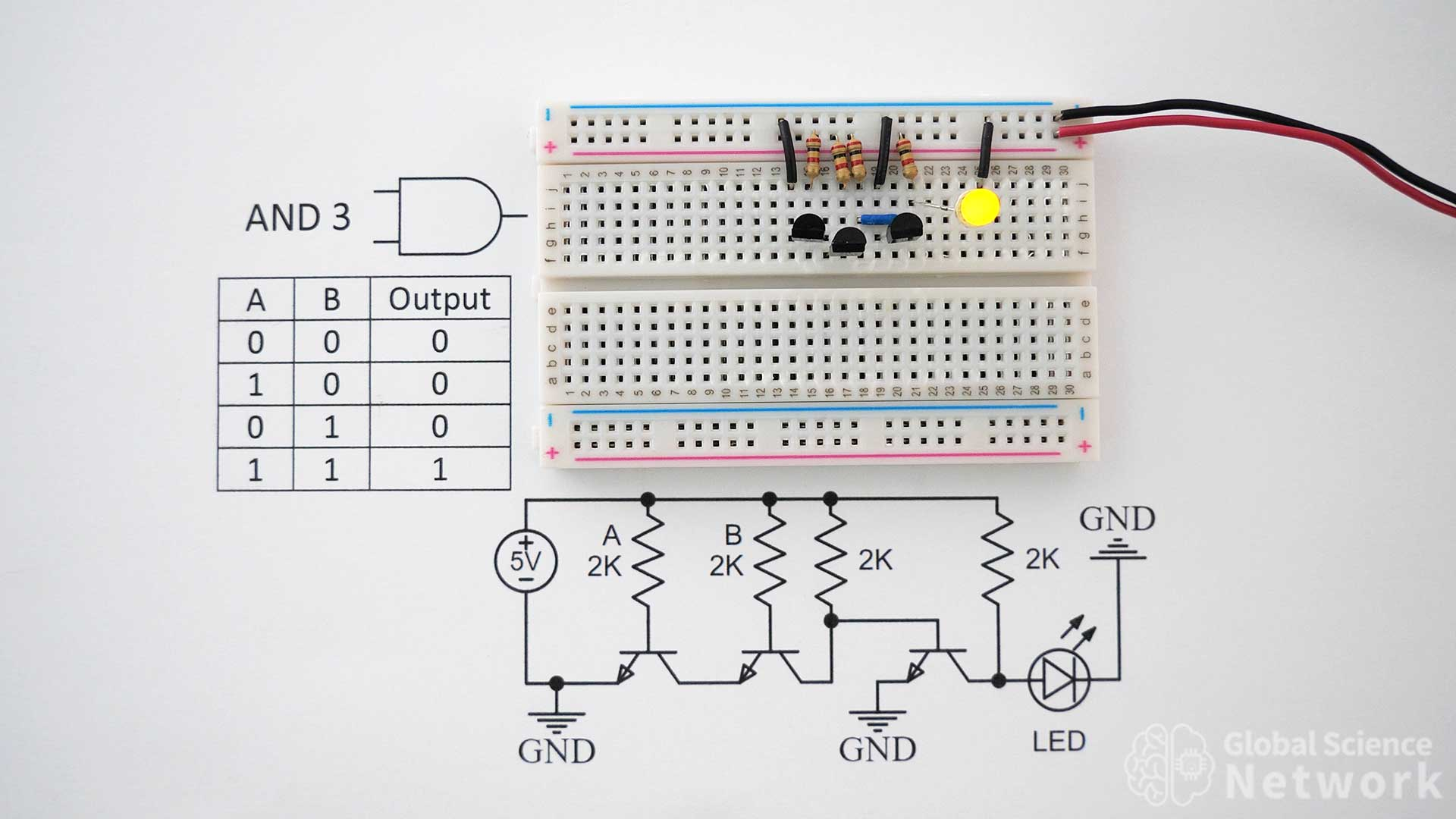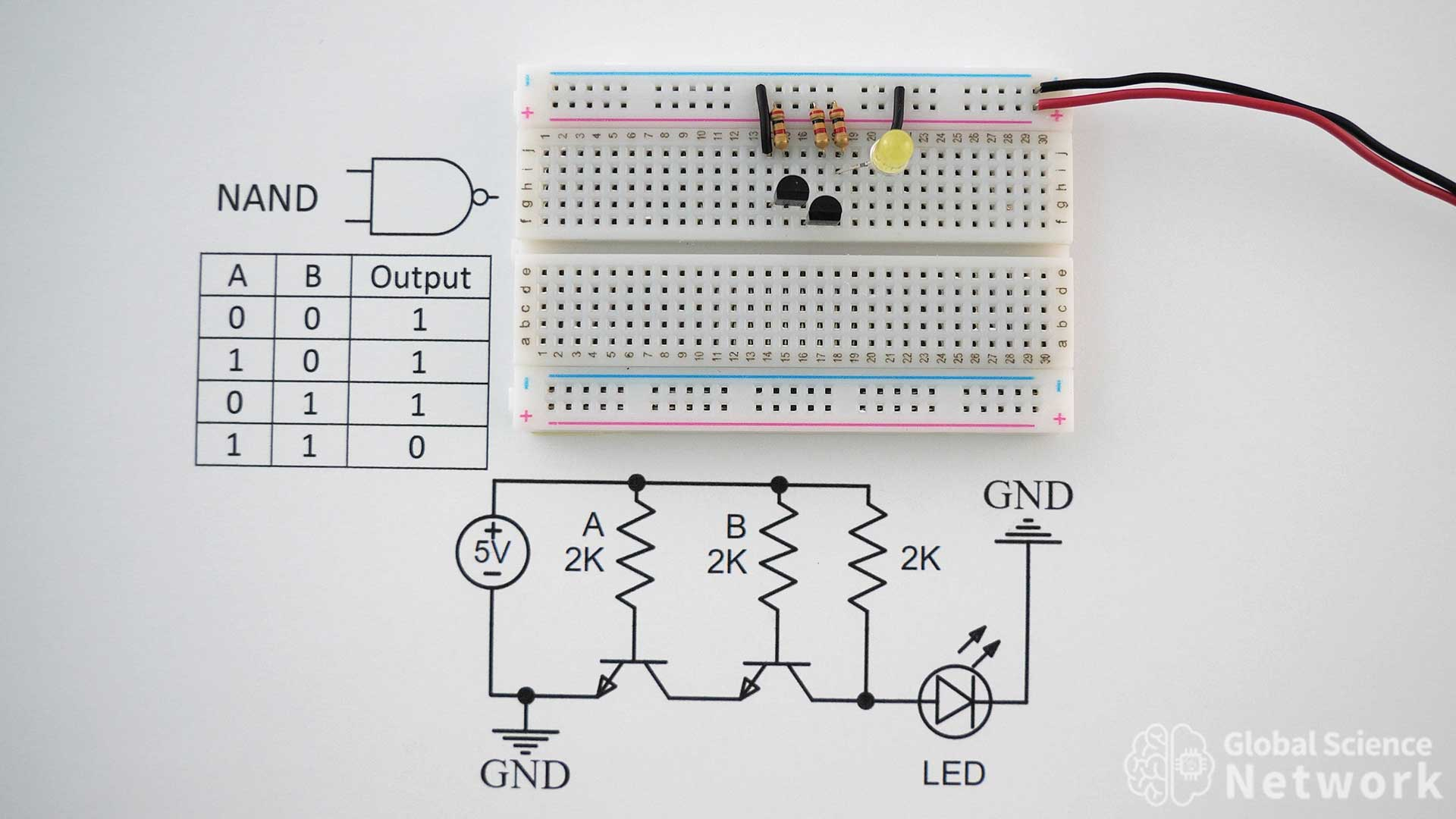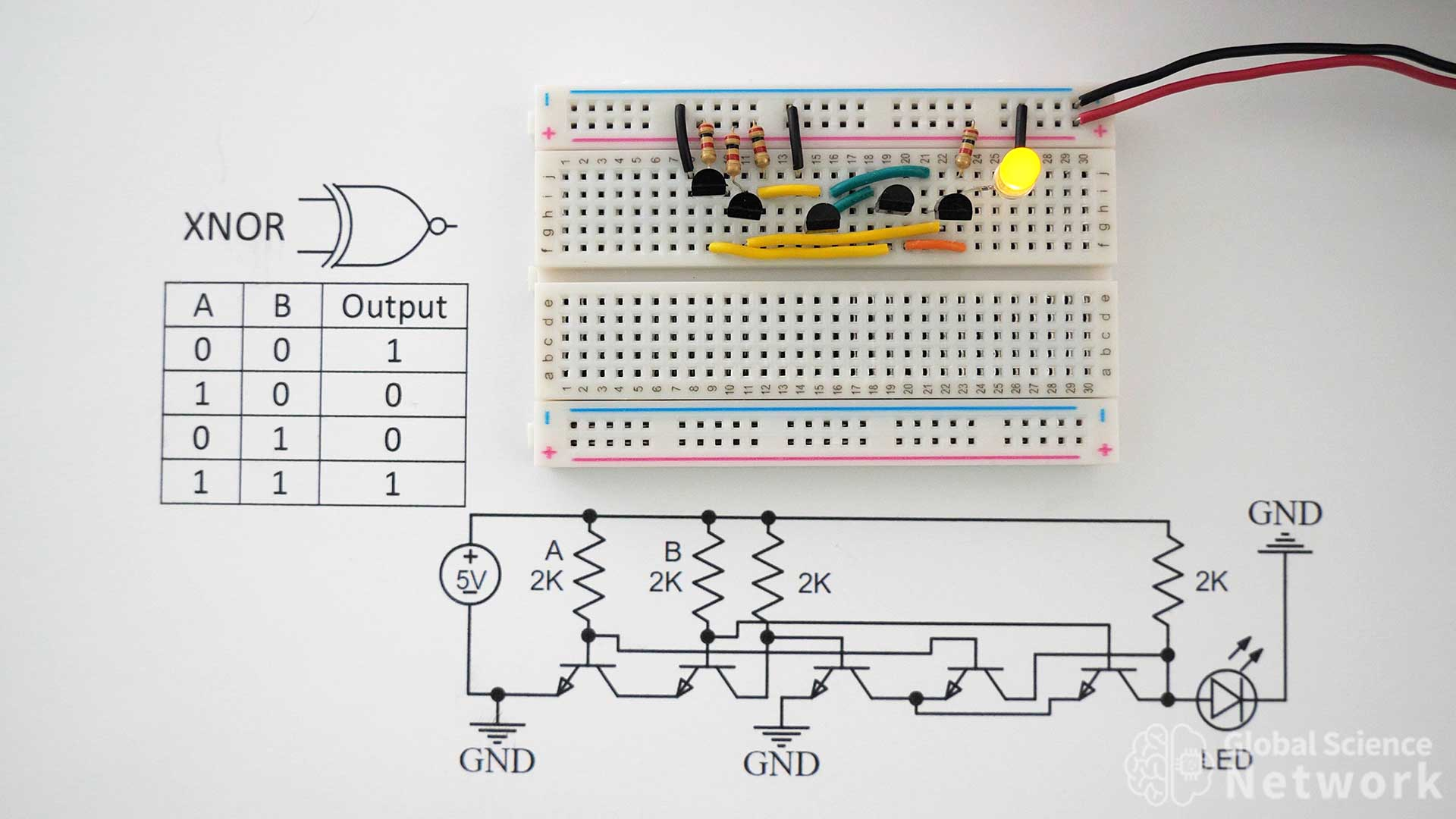# Digital Logic Gates

Digital logic gates are what allow a computer to perform computations using transistors. This is done by using the binary or base 2 number system. A transistor only has two states which are on or off. However, when multiple transistors are used together the output of the two inputs can be changed in a logical way.  This allows for AND, NAND, OR, NOR, XOR, and XNOR logic. Most logic gates can be made using multiple circuit configurations.The picture above shows all the types of logic gates built on breadboards using individual transistors. AND gate 6 and AND gate 7 use an integrated circuit that contains four AND gates. Many people understand the basics of digital logic gates but not enough to actually implement them at the transistor level. The logic gate needs to be wired in a configuration so the output can be sent further down the circuit. There are also more advanced configurations that allow for lower power consumption and faster switching time.

In the video above I discuss in detail how to build each type of logic gate on a breadboard using individual transistors, resistors, wires, and LEDs. I used consistent color with wires to make it clear how to wire the circuit. A circuit diagram is also provided so there is no ambiguity as to where to connect the wires. If you plan to build circuits using digital logic gates I recommend watching this video completely before starting. This will likely save you time in the long run.The name, symbol, and truth table for each logic gate is shown in the picture above. Often times circuits are not drawn at the transistor level and just the logic gate symbol is used. The truth table is very important as it makes it clear what the output should be based on the inputs. This is very useful when trying to build larger circuits such as the half adder, full adder, calculators, and computer processors.

## BufferA buffer is a simple circuit where the input value is the same as the output value. This means if the input is off the output will be off. If the input is on the output will be on. There are many reasons why a buffer may be added to the circuit. In most cases, it is to ensure a strong output signal. The input voltage or current may be a low value and running the input through the buffer helps ensure a consistent output level. There is more than one way to make a buffer so check out the buffer article for more information.

## InverterThe inverter uses one transistor to take an input signal and make the output signal the opposite. This means if the input is off the output will be on. If the input is on the output will be off. Many people say that a NAND gate is an AND gate with an inverter. These gates do have inverted logic but a NAND gate is not an AND gate with an inverter. In fact, the NAND gate can be made with two transistors and the AND gate requires three transistors when sending an output. The AND gate is basically a NAND gate with an inverter.  However, the LED in the circuit is also flipped as the current is flowing through the circuit in a two-transistor AND gate. The current is flowing toward the second ground and can be used as an output signal in a three-transistor AND gate.

## AND GateAn AND gate is only on when inputs A and B are both on. In all other cases, it is off. It can be made using many different transistor configurations. The case above uses three transistors. The first two transistors from the left are the inputs.  Right now both resistors are plugged in meaning the inputs are on. This makes it so the output is ON which is represented by the yellow LED. A and B are turned on by providing a positive voltage of over 0.6 volts into the base of the transistor.

## NAND GateThe NAND gate is a universal logic gate. This means that it can be used to make all the other types of logic gates. It is turned off when both inputs are on. In all other cases, it is turned on. In the photo, both inputs A and B are on which is why the LED is turned off. This is a simple logic gate that only requires two transistors. Using just two transistors it sends the output further down the circuit which is desired when incorporating the NAND logic gate into larger circuit designs.

If you want to see this NAND gate used in a larger circuit, check out the video above where I built a 4-bit computer on breadboards.

## OR GateThe OR gate is off when inputs A and B are both off. In all other cases, it is on. It is important to note that the OR gate is on during the AND condition. If you want the output to be on only when one of the inputs is on the exclusive OR gate is needed.  In the picture, both inputs A and B are on which is why the LED is on. The OR gate can also be made out of three NAND gates or by using two NOR gates. OR gate 2 shown above only requires three transistors and that is how I would recommend making an OR gate using individual transistors.

## NOR GateThe NOR gate is another universal logic gate. This means that it can make all the other types of logic gates. Like the NAND gate it also only requires two transistors. In the picture, the orange wire connects the collectors of both transistors. There is also ground going to the emitter of both transistors. This makes it so if any input is on the output will be off. The only case where the output will be on is when both inputs are off.

## XOR GateThe XOR gate is also called an exclusive OR gate. It is similar to an OR gate but is not on when both inputs A and B are on. In the picture, only input A is on which is why the LED is on. This is a common logic gate to see used to build half adders and full adders. It can be built in multiple configurations including using NAND and NOR gates. The one shown uses 6 transistors and the output can be sent to be used as inputs in other circuits.

## XNOR GateThe XNOR gate is also called the exclusive NOR gate. This means that it is not on when only input A or only input B is on. When both inputs are on or off the output will be on. This circuit only requires 5 transistors and the output can be sent to other circuits. In the photo inputs A and B are both on which is why the LED is lit up.